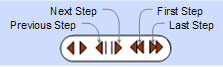# Isogonic or Jacobi's Theorem: Isogonals and Concurrent Point with Dynamic Geometry: TracenPoche Software. Level: High School, College, Mathematics Education.

 Proposition: Through vertices of a given triangle ABC, we draw two lines isogonal conjugates with respect to the corresponded angle. Prove that the lines connecting each vertex of the given triangle with the intersection point of the isogonal lines through the other vertices, AA', BB', and CC' are concurrent at one point J called the Jacobi Point. Interact with the figure below: Click the red button () on the figure to start the animation. Drag the red points to change the figure. Press P and click the left mouse button to start the step by step construction, use the next step button.      The isogonal conjugate line to BC' is the line BA' obtained by reflecting the line BC' on the angle bisector of angle B; in the figure above: angles ABC' = CBA'. Similarly AC' and AB' are isogonal conjugates (angles BAC' = CAB'), and CA' and CB' are isogonal conjugates (angles ACB' = BCA'). Interactive Geometry Software or Dynamic Geometry: You can alter the figure above dynamically in order to test and prove (or disproved) conjectures and gain mathematical insight that is less readily available with static drawings by hand. This page uses the TracenPoche dynamic geometry software and requires Adobe Flash player 7 or higher. TracenPoche is a project of Sesamath, an association of French teachers of mathematics. Instruction to explore the theorem above: Animation. Click the red buttonto start/stop animation Manipulate. Drag red points to change the figure. Step by Step construction. Press P and click the left mouse button on any free area to show the step-by-step bar and start the construction:Hide the step-by-step bar by using again the combination P + click left mouse.
 Home | Geometry | Dynamic Geometry | TracenPoche Index | Problems | Triangles | Isogonal Lines | Concurrent lines | Email | By Antonio Gutierrez